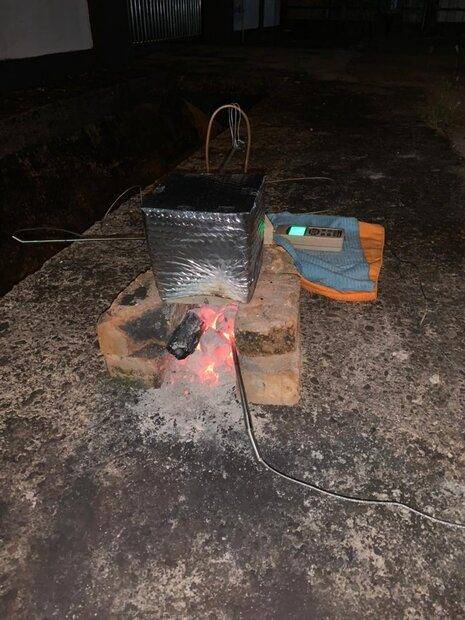# Heat Transfer Formulae for Charcoal-Concrete Experiment

• ahbin98
In summary, the experiment procedure involved using burning charcoal as the heat source and three thermocouples to measure the temperature of the charcoal, the inner temperature of the concrete block, and the top surface temperature of the concrete block. After obtaining the data, it was discovered that the formula Q=mcΔT was not suitable for calculating the heat transfer from the charcoal to the internal part of the concrete and from the internal part of the concrete to the top surface. The use of this formula resulted in illogical data, with the first set having a greater heat coefficient despite the second set having a higher internal concrete temperature. The recommendation to use Finite Element Analysis and consult the book "Fundamentals of Heat and Mass Transfer" for suitable formulas is alsof

#### ahbin98

TL;DR Summary
I am doing my final year project regarding how well can concrete block absorb heat and trap the thermal energy within itself and save it for later use which is thermal energy storage.
My experiment procedure is that I am using burning charcoal as the heat source, and there are three thermocouples used, first one measuring the temperature of the charcoal, second one measuring the inner temperature of the concrete block, third thermocouple is placed on the top part which is furthest from the heat source.Now I've obtained the experiment data, but I can't figure out which formulae should I use to process the data, at first I thought using the Q=mcΔT is ok, but in the end I realized that is not a suitable equation for me to use. I am searching for a formulae which allows me to calculate the heat transfer from charcoal to the internal part of the concrete, and the heat transfer from the internal part of the concrete to the top surface part of the concrete.
If I use Q=mcΔT, let's assume, the charcoal have a temperature of 700°C (T0), the internal of concrete have a temperature of 50°C (T1), compared to another set of data, where charcoal have a temperature of 700°C (T0), and internal of concrete have a temperature of 100°C (T1). If these 2 sets of data applied to the formulae Q=mcΔT, the first set will have greater heat coefficient since T0-T1 is greater than the second set of T0-T1, which seems illogical since the second set should have greater heat coefficient as the temperature of the internal part of the concrete rise higher. This makes me think I am using the wrong formulae, are there any other formulae suitable for my experimental data? Please send helps and thank you.

Have you considered using Finite Element Analysis to get a solution of this problem and compare the results with experiment ?

For formulas check the "Fundamentals of Heat and Mass Transfer" by Incropera. It covers lots of different cases with practical examples.

•berkeman